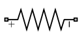# Resistor

Model resistor for circuit envelope analysis

•Libraries:
RF Blockset / Circuit Envelope / Elements

## Description

The Resistor block models a resistor within the RF Blockset™ circuit envelope simulation environment. For an introduction to RF simulation, see the example, Simulate High Frequency Components.

The block implements the relation

`$v\left(t\right)=Ri\left(t\right)$`

where:

• R represents the resistance, as a function of temperature.

• i(t) represents the current through the capacitor at time t.

• v(t) represents the voltage across the terminals of the capacitor at time t.

RF Blockset current and voltage signals consist of in-phase (Ik) and quadrature (Qk) components at each frequency fk specified in the Configuration block:

`$\begin{array}{c}i\left(t\right)=\sum _{\left\{{f}_{k}\right\}}\left({i}_{{I}_{k}}\left(t\right)+j\cdot {i}_{{Q}_{k}}\left(t\right)\right){e}^{j\left(2\pi {f}_{k}\right)t}\\ v\left(t\right)=\sum _{\left\{{f}_{k}\right\}}\left({v}_{{I}_{k}}\left(t\right)+j\cdot {v}_{{Q}_{k}}\left(t\right)\right){e}^{j\left(2\pi {f}_{k}\right)t}\end{array}$`

Resistor block mask icons are dynamic and indicate the current state of the applied noise parameter. This table shows you how the icons on this block vary based on the state of the Simulate noise parameter on the block.

Simulate noise: onSimulate noise: offTo learn how you can use the Resistor block to design output matching network, open RF Receiver Front End subsystem and select LinearOutput Matching in Radar System Modeling example.

## Parameters

expand all

Resistance value , specified as a real number in ohms greater than zero. Specify the units of the resistance from the corresponding drop-down menu.

Select this parameter, to simulate thermal noise in a resistor. Then, in the Configuration block dialog box, also select the Simulate noise check box. By default, both Simulate noise check boxes are selected.

This parameter inserts a current noise source with the single-sided power density of 4 kT/R A2/Hz, where:

• k is the Boltzmann constant

• T is the value of the Temperature parameter, in degrees Kelvin. (Also located in the Configuration block.)

expand all